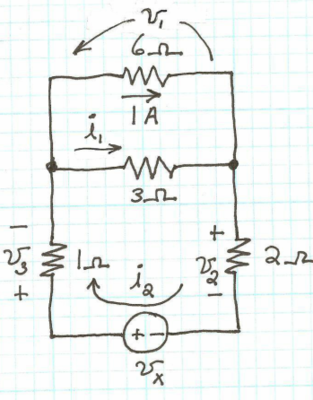## Electrical Engineering: Principles & Applications (6th Edition)

Published by Prentice Hall

# Chapter 1 - 1.7 - Problems - Introduction to Circuits - Page 40: P1.66

#### Answer

a. the 1 and 2 ohm resistors and the voltage source are in series. b. the 3 and 6 ohm resistors are in parallel. c. $v_x=15V$

#### Work Step by Step

c. Voltage across 6 ohm resistor is 6V, which will also be voltage across 3 ohm resistor. Current through 3 ohm resistor will then be 2A. Using KCL we add the currents to find that $i_2=3A$ and then apply Ohm's law again to find that the voltage across the 1 ohm resistor is 3 volts and across the 2 ohm resistor is 6 volts. Apply KVL to find that the voltage source is equivalent to the sum of the voltage drops across all series components (6 and 3 ohm resistors will only be counted once) and that $v_x=15V$After you claim an answer you’ll have 24 hours to send in a draft. An editor will review the submission and either publish your submission or provide feedback.